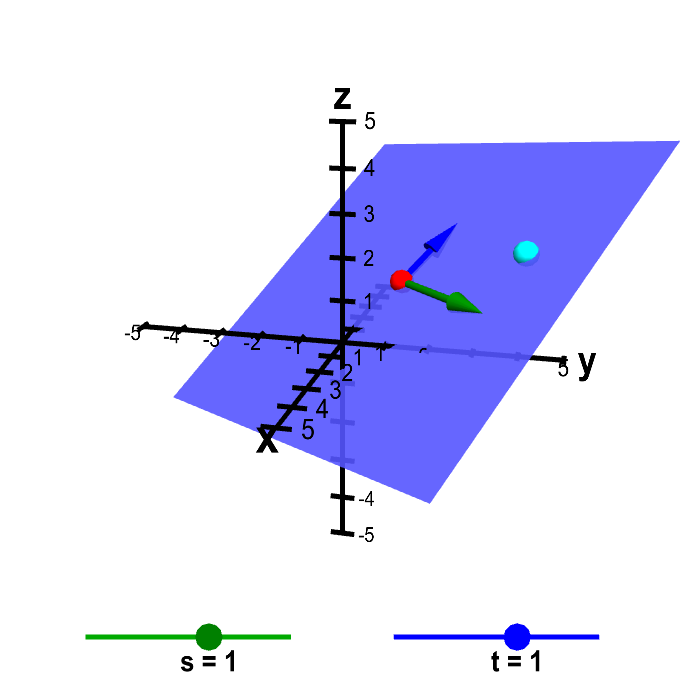# Math Insight

### Applet: Parametrization of a planeThe plane is determined by the point $\color{red}{\vc{p}}$ (in red) and the vectors $\color{green}{\vc{a}}$ (in green) and $\color{blue}{\vc{b}}$ (in blue), which you can move by dragging with the mouse. The point $\vc{x} = \vc{p} + s\vc{a} + t \vc{b}$ (in cyan) sweeps out all points in the plane as the parameters $s$ and $t$ sweep through their values. You can move the cyan point $\vc{x}$ either by changing the values of $s$ and $t$ with the sliders or by moving the point directly (which updates the values of $s$ and $t$). For this applet, the values of $s$ and $t$ are restricted to be between -5 and 5, which restricts the possible locations of the cyan point, depending on the values of $\vc{a}$ and $\vc{b}$.